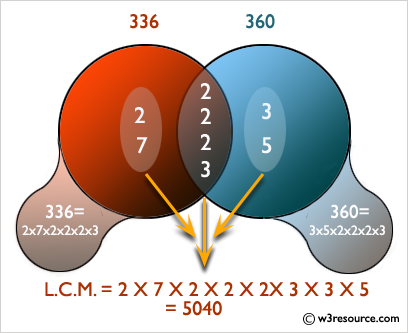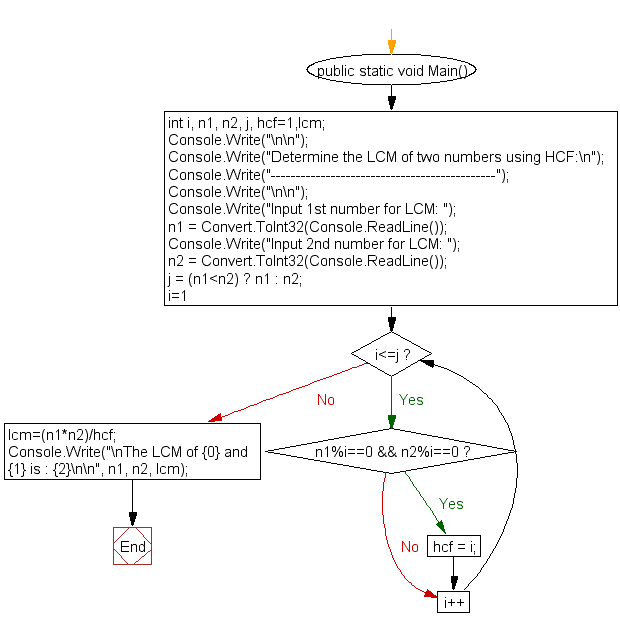﻿ C# - Determine the LCM of two numbers using HCF - w3resource# C# Sharp Exercises: Determine the LCM of two numbers using HCF

## C# Sharp For Loop: Exercise-44 with Solution

Write a program in C# Sharp to find LCM of any two numbers using HCF.Sample Solution:-

C# Sharp Code:

``````using System;
public class Exercise44
{
public static void Main()
{
int i, n1, n2, j, hcf=1,lcm;
Console.Write("\n\n");
Console.Write("Determine the LCM of two numbers using HCF:\n");
Console.Write("---------------------------------------------");
Console.Write("\n\n");

Console.Write("Input 1st number for LCM: ");
Console.Write("Input 2nd number for LCM: ");

j = (n1<n2) ? n1 : n2;

for(i=1; i<=j; i++)
{

if(n1%i==0 && n2%i==0)
{
hcf = i;
}
}
/* We know  the multiplication of HCF and LCM is equivalant
to the multiplication of these two numbers.*/
lcm=(n1*n2)/hcf;

Console.Write("\nThe LCM of {0} and {1} is : {2}\n\n", n1, n2, lcm);
}
}
```
```

Sample Output:

```Determine the LCM of two numbers using HCF:
---------------------------------------------
Input 1st number for LCM: 8
Input 2nd number for LCM: 12
The LCM of 8 and 12 is : 24
```

Flowchart:C# Sharp Code Editor:

Contribute your code and comments through Disqus.

What is the difficulty level of this exercise?

Test your Programming skills with w3resource's quiz.

﻿